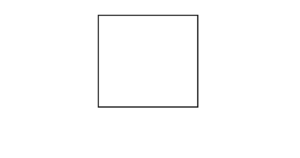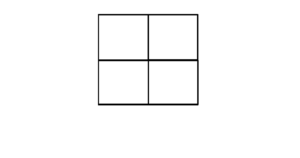# Find the number of squares inside the given square grid

Given a grid of side N * N, the task is to find the total number of squares that exist inside it. All squares selected can be of any length.

Examples:

Input: N = 1
Output: 1Input: N = 2
Output: 5Input: N = 4
Output: 30

## Recommended: Please try your approach on {IDE} first, before moving on to the solution.

Approach 1: Taking a few examples, it can be observed that for a grid on size N * N, the number of squares inside it will be 12 + 22 + 32 + … + N2

Below is the implementation of the above approach:

## C++

 // C++ implementation of the approach  #include  using namespace std;     // Function to return the number  // of squares inside an n*n grid  int cntSquares(int n)  {      int squares = 0;      for (int i = 1; i <= n; i++) {          squares += pow(i, 2);      }      return squares;  }     // Driver code  int main()  {      int n = 4;         cout << cntSquares(4);         return 0;  }

## Java

 // Java implementation of the approach  class GFG {         // Function to return the number      // of squares inside an n*n grid      static int cntSquares(int n)      {          int squares = 0;          for (int i = 1; i <= n; i++) {              squares += Math.pow(i, 2);          }          return squares;      }         // Driver code      public static void main(String args[])      {          int n = 4;             System.out.print(cntSquares(4));      }  }

## Python3

 # Python3 implementation of the approach      # Function to return the number   # of squares inside an n*n grid   def cntSquares(n) :          squares = 0;       for i in range(1, n + 1) :          squares += i ** 2;          return squares;      # Driver code   if __name__ == "__main__" :          n = 4;          print(cntSquares(4));      # This code is contributed by AnkitRai01

## C#

 // C# implementation of the approach  using System;     class GFG   {         // Function to return the number      // of squares inside an n*n grid      static int cntSquares(int n)      {          int squares = 0;          for (int i = 1; i <= n; i++)           {              squares += (int)Math.Pow(i, 2);          }          return squares;      }         // Driver code      public static void Main(String []args)      {          int n = 4;             Console.Write(cntSquares(n));      }  }     // This code is contributed by 29AjayKumar

Output:

30


Approach 2: By the use of direct formula.
However, the sumhas the closed form (direct formula). Hence, we can employ this to calculate the sum intime.

Below is the implementation of the above approach:

## C++

 // C++ implementation of the approach  #include     using namespace std;     int cnt_squares (int n)  {      /* Function to return the number       of squares inside an n*n grid */        return n * (n + 1) * (2 * n + 1) / 6;  }     // Driver code  int main()  {      cout << cnt_squares (4) << endl;         return 0;  }

## Java

 // Java implementation of the approach  class GFG {      static int cntSquares (int n) {          /* Function to return the number          of squares inside an n*n grid */                return n * (n + 1) * (2 * n + 1) / 6;      }         // Driver code      public static void main(String args[]) {          System.out.println (cntSquares(4));      }  }

## Python3

 # Python3 implementation of the approach      """  Function to return the number   of squares inside an n*n grid   """    def cntSquares(n) :       return int (n * (n + 1) * (2 * n + 1) / 6)     # Driver code   if __name__ == "__main__" :       print (cntSquares (4));

## C#

 // C# implementation of the approach  using System;     class GFG   {         /* Function to return the number       of squares inside an n*n grid */     static int cntSquares (int n)      {          return n * (n + 1) * (2 * n + 1) / 6;      }         // Driver code      public static void Main (String[] args)      {          Console.Write (cntSquares (4));      }  }

Output:

30


Attention reader! Don’t stop learning now. Get hold of all the important DSA concepts with the DSA Self Paced Course at a student-friendly price and become industry ready.

My Personal Notes arrow_drop_upCheck out this Author's contributed articles.

If you like GeeksforGeeks and would like to contribute, you can also write an article using contribute.geeksforgeeks.org or mail your article to contribute@geeksforgeeks.org. See your article appearing on the GeeksforGeeks main page and help other Geeks.

Please Improve this article if you find anything incorrect by clicking on the "Improve Article" button below.

Article Tags :
Practice Tags :

1

Please write to us at contribute@geeksforgeeks.org to report any issue with the above content.